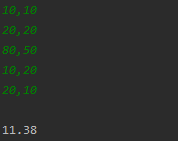# 蓝桥杯 平面点集中4点最小平均距离

10,10
20,20
80,50
10,20
20,10



11.38


• 写回答

#### 2条回答默认 最新

•pzzhao 2023-03-06 20:20
关注

该回答引用自ChatGPT
先贴本人本地执行结果这个是我根据chatGPT代码修改的。

最近感觉chatGPT给的代码经常有问题

import java.util.*;
public class Main {
public static void main(String[] args) {
Scanner sc = new Scanner(System.in);
double min = Integer.MAX_VALUE;
//先将点存储在Point数组里面
ArrayList<Point> list = new ArrayList<>();
String line = "";
while (!("").equals(line=sc.nextLine())) {
String[] s = line.split(",");
}
//穷举所有四个点的组合
for (int i = 0; i < list.size(); i++) {
for (int j = i + 1; j < list.size(); j++) {
for (int k = j + 1; k < list.size(); k++) {
for (int l = k + 1; l < list.size(); l++) {
double sum = 0;
//计算平均距离
sum += getDistance(list.get(i), list.get(j));
sum += getDistance(list.get(i), list.get(k));
sum += getDistance(list.get(i), list.get(l));
sum += getDistance(list.get(j), list.get(k));
sum += getDistance(list.get(j), list.get(l));
sum += getDistance(list.get(k), list.get(l));
sum /= 6;
if (sum < min) {
min = sum;
}
}
}
}
}
System.out.printf("%.02f", min);
}
//计算两点之间距离
public static double getDistance(Point a, Point b) {
return Math.sqrt(Math.pow(a.x - b.x, 2) + Math.pow(a.y - b.y, 2));
}
}
class Point {
int x;
int y;
public Point(int x, int y) {
this.x = x;
this.y = y;
}
}


本回答被题主选为最佳回答 , 对您是否有帮助呢?
评论

#### 悬赏问题

• ¥15 k210显示failed init to model
• ¥15 Evil-droid生成的APK手机已经下载但无法建立任务
• ¥25 c语言韩信点兵的变式
• ¥15 怎么根据书上的例子完成这个问题呢？
• ¥15 ECharts 增加Zoom,整行包括右边的Text一起滑动
• ¥15 关于网上一个easyx制作的见缝插针小游戏(c++)
• ¥15 开地址法双散列函数处理碰撞
• ¥15 想问一下这个是什么情况 虚拟机Linux打不开了
• ¥15 联通光猫掉注册了怎么重新注册上去
• ¥15 关于unity开发steamvr程序遇到的问题